+ +

# Zernike polynomials

## The Zernike polynomials

### A wavefront error can be plotted with a color scale (micron step units), on a domain which is the exit pupil disk.In the ANSI recommended polar coordinates system representation (2D system where the distance and angle of any point on the plane is calculated from the reference point and direction), the wavefront error depicts the optical path difference OPD (in microns) from the reference surface. The above representation corresponds to the OPD with a flat wavefront (green level). Each color step represents a phase shift of 1 micron. The zero level is the « mean » of the wavefront, which is the horizontal plane that separates in two equal components – the relatively advanced and delayed phases. The « height » of that zero level mean with regards to the lowest point of the wavefront error corresponds to the RMS value of the first Zernike term, named piston (Z00).

## The Zernike pyramid

### The Zernike modes are usually presented as a pyramid: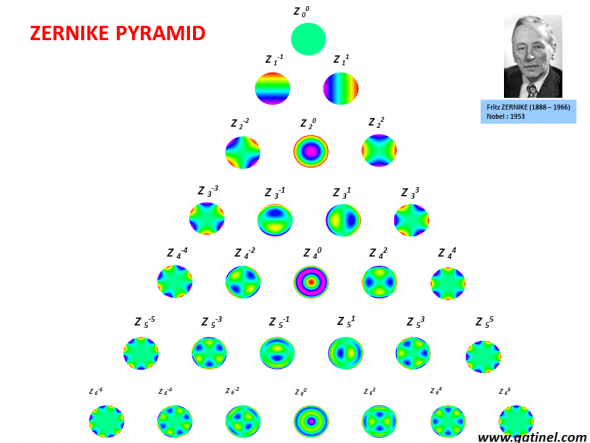Representation of the Zernike pyramid formed by the first 28 modes (the Zernike pyramid has an infinite number of modes). The mean value of each mode (except for the piston) is zero. Think of each mode (or harmonic) as a kind of particular wavefront error within the pupil disk. Some modes, such as defocus (Z 20), are familiar to the clinician, whereas other (ex: tetrafoil : Z 4-4) are not.

## The Zernike polynomials analytical expression

5. ### m is a negative or positive integer called the « azimuthal » or « angular frequency ».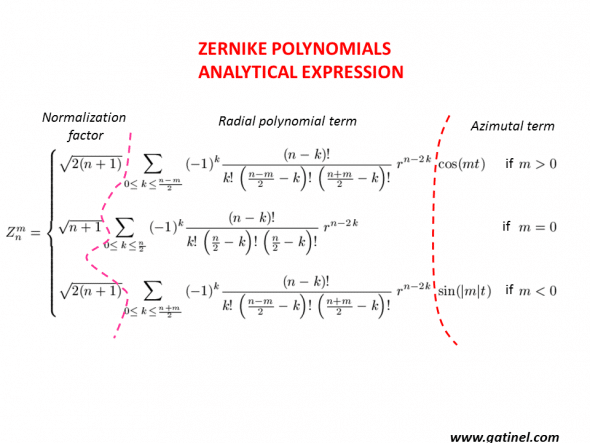To obtain a particular Zernike mode of « n » radial degree and « m » azimuthal frequency, just insert the actual values of n and m in the equations. There is an important relation between n and m, which is conveyed by another variable « k », which is an integer comprised between zero and half of the sum or half of the difference between n and m. Because of these relations, the difference between the absolute values of n and m cannot be 1, or any odd number. Hence, there are No Z 10 or Z 4-3 Zernike polynomials. When m = 0, there is no angular dependence and the mode is « rotationally symmetrical » eg. Defocus and Spherical aberration. Of course, regular astigmatism is azimuthally variable (m=2). The normalization factor is relatively straightforward to calculate, it just depends on n. It is a scaling factor, which makes the RMS of each mode equal to one on the unit pupil (radius = 1). It is important to note that the radial polynomial component can be constituted of the sum of terms of various radial degree. Not all the Zernike modes are « pure » in their radial degree (more on this in our newly published paper).

n corresponds to the « degree » of a given mode. When n ≤ 2, the mode is said to be of a « lower » degree or LOA (Lower order aberrations).

Conversely, modes in which n ≥ 3 are said to be of a « higher » degree or HOA (Higher order aberrations).

Classically, spectacle corrected aberrations (myopia, hyperopia, and astigmatism) correspond to Zernike polynomials of radial degree n=2.

Let us focus on a Zernike mode named trefoil: Z 3-3. It may help the reader to mentally connect the equation with the shape of the mode.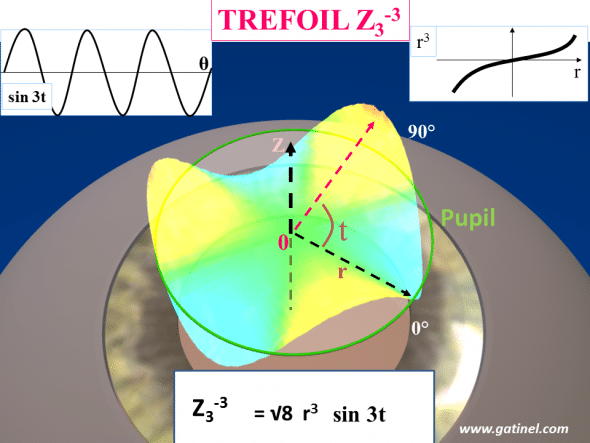Representation of triangular astigmatism (trefoil) with the Z 3-3 polynomial on the normalized unit pupil disk (green ring). The surface of this mode is equal to the product of a 3rd order polynomial radial term (r3) where r is the radial distance from the pupil center, and a trigonometric function with an azimuthal frequency of 3 (sin3t), where t corresponds to the angle formed with the horizontal line (as for the common astigmatism axis angle plot). A normalization factor is a scaling number which makes each mode having a total RMS of unity. If you would like to visualize each mode as a vector, the normalization factor makes this vector a « unit vector », having a norm equal to one.

### This is another Zernike mode called « secondary astigmatism« :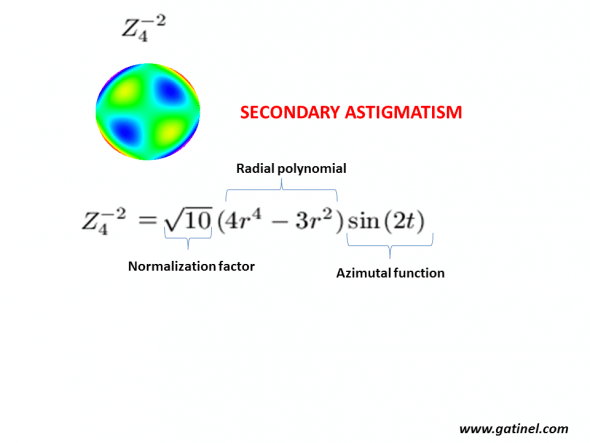Example of a non-radially symmetrical Zernike mode (secondary astigmatism). This mode is formed by the product of a normalization factor, a radial polynomial (a function of r) and an azimuthal function (a function of t). Note that the radial polynomial is not « pure » in r4, but contains also lower order azimuthal defocus (in r2). This explains the « tormented » shape of this mode. The presence of low order terms in the radial polynomial of some high order modes is due to the necessity of ensuring orthogonality with modes of lower radial degree but identical azimuthal frequency. It can cause artifacts in the low order wavefront coefficients’value.

### The primary spherical aberration Z 40 is a « famous » Zernike mode: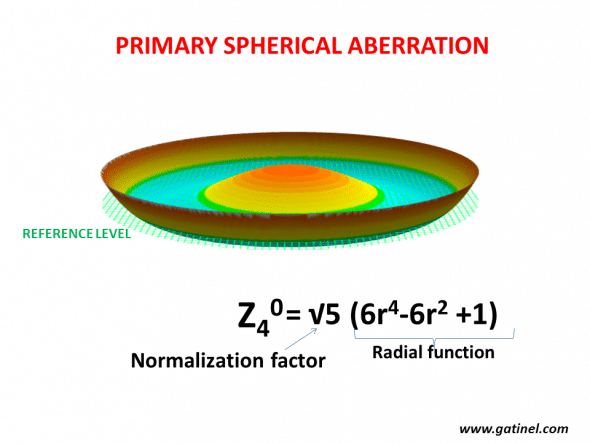Example of a radially symmetrical Zernike mode (primary spherical astigmatism). This mode is formed by the product of a normalization factor, a radial polynomial (a function of r) but no azimuthal function; it is rotationally symmetrical. Note that the radial polynomial function is not « pure » in r4, but contains also lower order defocus (in r2)

### The structure of the Zernike pyramid is based on the values of n and m: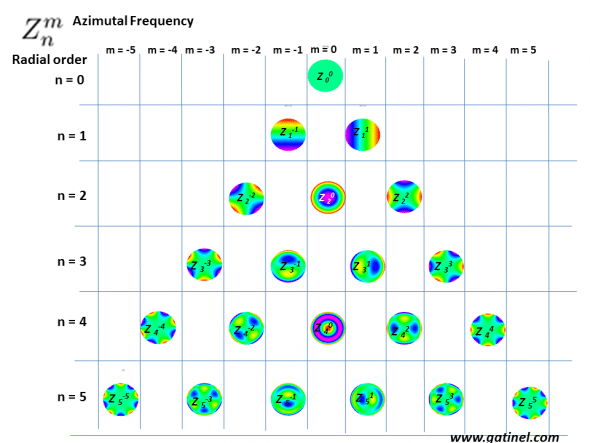In the Zernike pyramid, the position of each polynomial depends on its radial order and azimuthal frequency.

### Here follows the analytical expression of the first Zernike modes in radial coordinates: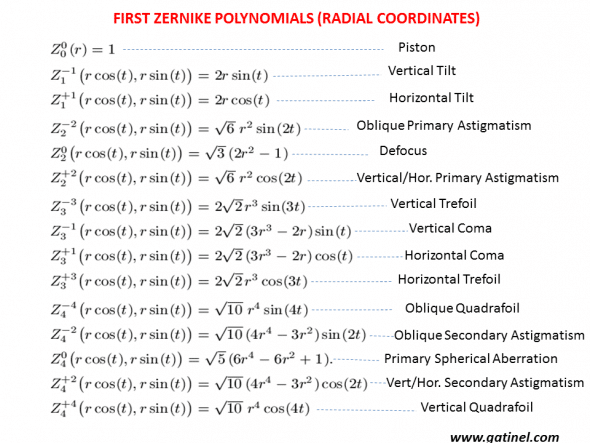## Sampling the wavefront error into Zernike modes

### A wavefront error can be sampled, or decomposed, into Zernike modes; each RMS coefficient corresponds to the weight of the mode.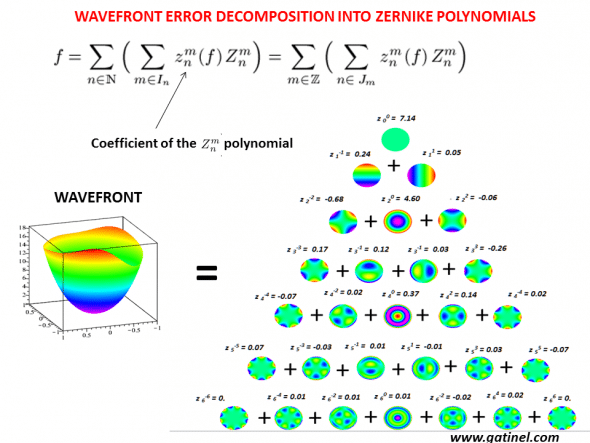The total wavefront surface error (left) is equal to the sum of all the non zero RMS coefficients in the Zernike mode. The RMS value of this specific wavefront error is equal to the square root of the sum of all the squared coefficients.

## Example:

### This is an example of a wavefront reconstruction obtained with the wavefront sensor OPDscan III (Nidek)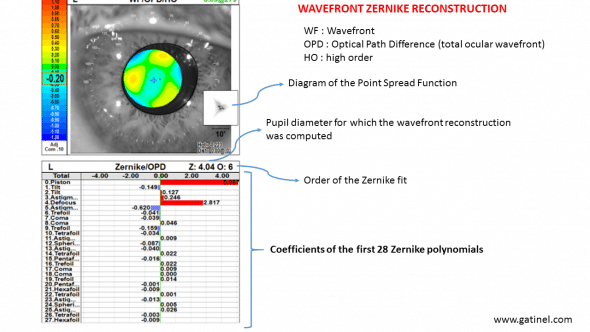The wavefront sensor acquires optical data using a slit-scanning automated retinoscopy

#### See other relevant examples and material :

Novel wavefront decomposition method

Pre and Post LASIK aberrations

PresbyLASIK

Other examples

Wavefront sensing